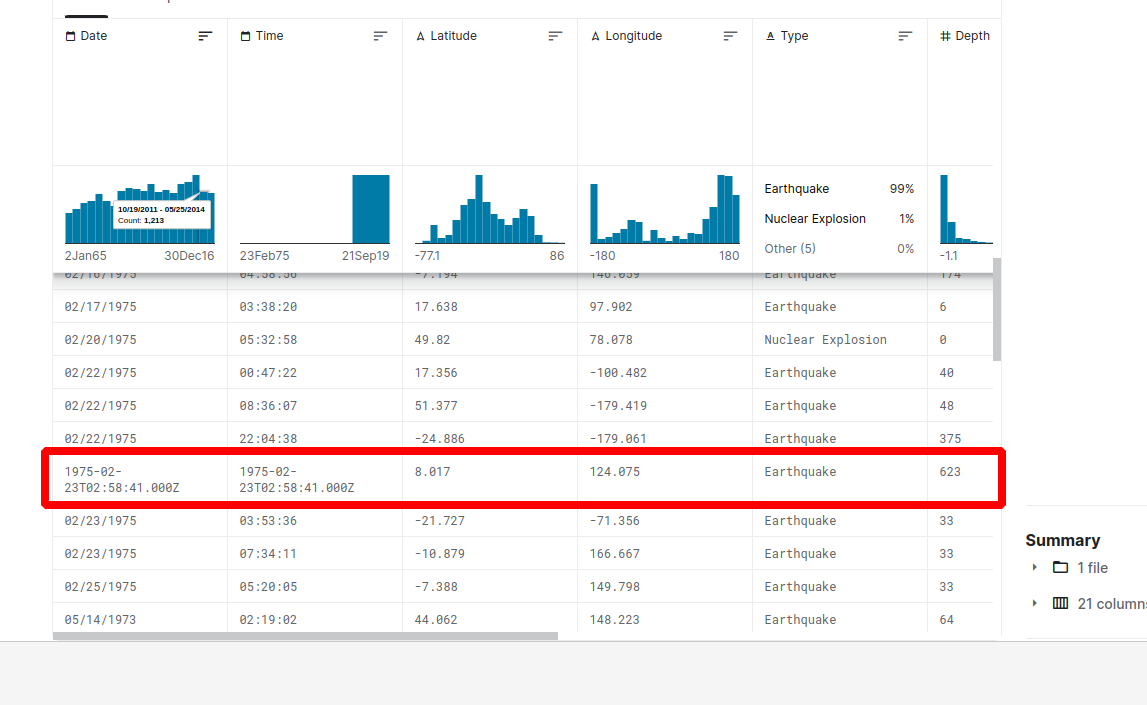In this article we will see how to solve errors related to `pd.to_datetime()` in Pandas.

Typical errors for datetime conversion in Pandas are:

• `ParserError: Unknown string format: 27-02-2022 sdsd6:25:00 PM`
• `AttributeError: Can only use .dt accessor with datetimelike values`
• `ValueError: only leading negative signs are allowed`
• `ValueError: time data '1975-02-23T02:58:41.000Z' does not match format '%H:%M:%S' (match)`

This could be due to:

• mixed dates and time formats in single column
• wrong date format
• datetime conversion with errors

## Setup

In this tutorial we will work with this dataset from Kaggle: earthquake-database.

As we can see from the image above there are errors related to mixed formats in the dataset:## Detect wrong dates in Pandas - errors='coerce'

First we will try to detect the wrong date of time format by using the date parameter - `errors='coerce'`.

So after looking at the data we know that column "Time" has the following format: `'%H:%M:%S'`.

Now we can try to parse all times in this column and extract only the ones which are parsed with errors:

``````df[pd.to_datetime(df['Time'], format='%H:%M:%S', errors='coerce').isna()]['Time']
``````

the result is 3 rows with wrong format:

``````3378     1975-02-23T02:58:41.000Z
7512     1985-04-28T02:53:41.530Z
20650    2011-03-13T02:23:34.520Z
Name: Time, dtype: object
``````

## Detect mixed time formats - str.len()

Let's see how to detect problematic values in the DateTime column of Pandas DataFrame. We can find the wrong date format by using string methods.

We will count the length of each date time:

``````df['Time'].str.len().value_counts()
``````

the results show us 3 problematic rows:

``````8     23409
24        3
Name: Time, dtype: int64
``````

To extract the wrong or different ones we can do:

``````df[df['Time'].str.len() > 8]['Time']
``````

Which will give us:

``````3378     1975-02-23T02:58:41.000Z
7512     1985-04-28T02:53:41.530Z
20650    2011-03-13T02:23:34.520Z
Name: Time, dtype: object
``````

To exclude rows with different rows we can do:

``````df = df[df['Date'].str.len() == 8]
``````

This way is better for for working with date or time formats like:

• HH:MM:SS
• dd/mm/YYYY

## Infer date and time format from datetime

This option is best when we need to work with formats which has date and time like:

• '%Y-%m-%dT%H:%M:%S.%f'
• '%Y-%m-%dT%H:%M:%S'

First we will find the most frequent format in the column by:

``````import numpy as np
from pandas.core.tools.datetimes import _guess_datetime_format_for_array
array = np.array(df["Date"].to_list())
_guess_datetime_format_for_array(array)
``````

This would give us:

``````'%m/%d/%Y'
``````

Next we will try to convert the whole column with this format:

``````pd.to_datetime(df["Date"], format='%m/%d/%Y')
``````

This will result into error:

``````ValueError: time data '1975-02-23T02:58:41.000Z' does not match format '%m/%d/%Y' (match)
``````

Now we can exclude rows with this format or convert them with different format:

### Semantic date errors in Pandas

Sometimes there isn't a code error but the date is wrong. This is when there are parsing errors. For example day and month are wrongly inferred or there are two date formats. To find more to this problem check:

How to Fix Pandas to_datetime: Wrong Date and Errors

### Convert string to datetime

If you want to find more about convert string to datetime and infer date formats you can check: Convert String to DateTime in Pandas